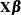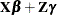## Specification and Parameterization of Model Effects

Subsections:

High-performance statistical procedures that have a MODEL statement support the formation of effects. An effect is an element in a linear model structure that is formed from one or more variables. At some point the statistical representations of these models involve linear structures such asorThe model matricesandare formed according to effect construction rules.

Procedures that also have a CLASS statement support the rich set of effects that is discussed in this section. In order to correctly interpret the results from a statistical analysis, you need to understand how construction (parameterization) rules apply to regression-type models, whether these are linear models in the HPREG procedure or generalized linear models in the HPLOGISTIC and HPGENSELECT procedures.

Effects are specified by a special notation that uses variable names and operators. There are two types of variables: classification (or CLASS) variables and continuous variables. Classification variables can be either numeric or character and are specified in a CLASS statement. For more information, see the section Levelization of Classification Variables. An independent variable that is not declared in the CLASS statement is assumed to be continuous. For example, the heights and weights of subjects are continuous variables.

Two primary operators (crossing and nesting) are used for combining the variables, and several additional operators are used to simplify effect specification. Operators are discussed in the section Effect Operators.

High-performance statistical procedures that have a CLASS statement support a general linear model (GLM) parameterization and a reference parameterization for the classification variables. The GLM parameterization is the default for all high-performance statistical procedures. For more information, see the sections GLM Parameterization of Classification Variables and Effects and Reference Parameterization.